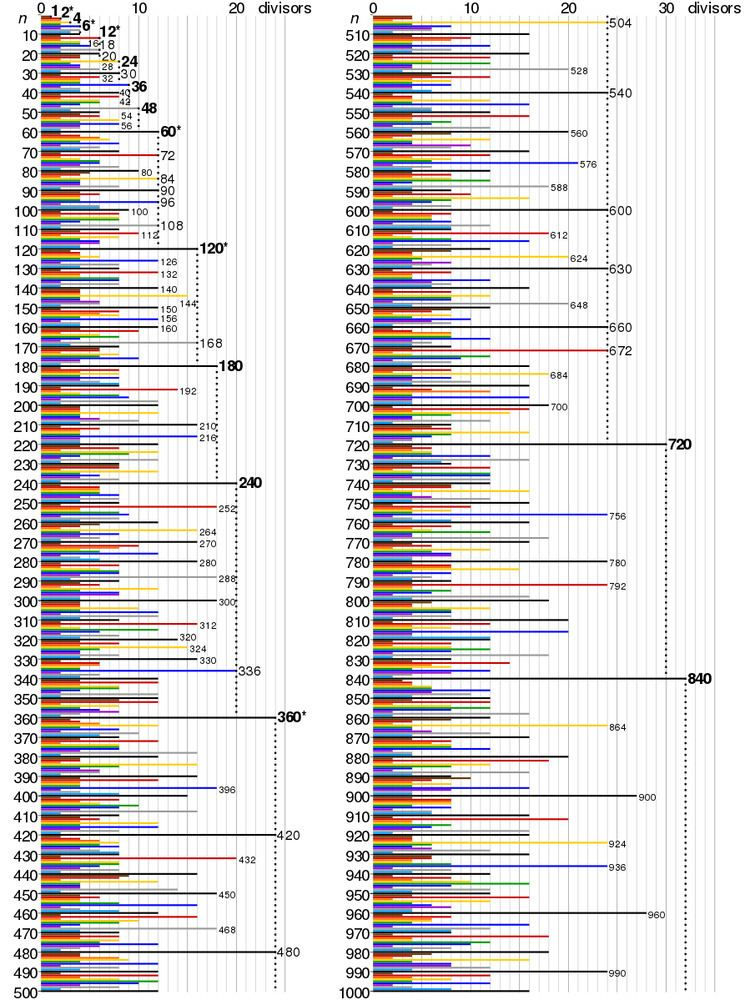# Highly composite number

Updated onA highly composite number (HCN) is a positive integer with more divisors than any smaller positive integer has. The term was coined by Ramanujan (1915). However, Jean-Pierre Kahane has suggested that the concept might have been known to Plato, who set 5040 as the ideal number of citizens in a city as 5040 has more divisors than any numbers less than it.

## Contents

The related concept of largely composite number refers to a positive integer which has at least as many divisors as any smaller positive integer.

## Examples

The initial or smallest 38 highly composite numbers are listed in the table below (sequence A002182 in the OEIS). The number of divisors is given in the column labeled d(n).

The table below shows all the divisors of one of these numbers.

The 15,000th highly composite number can be found on Achim Flammenkamp's website. It is the product of 230 primes:

a 0 14 a 1 9 a 2 6 a 3 4 a 4 4 a 5 3 a 6 3 a 7 3 a 8 2 a 9 2 a 10 2 a 11 2 a 12 2 a 13 2 a 14 2 a 15 2 a 16 2 a 17 2 a 18 2 a 19 a 20 a 21 a 229 ,

where a n is the sequence of successive prime numbers, and all omitted terms (a22 to a228) are factors with exponent equal to one (i.e. the number is 2 14 × 3 9 × 5 6 × × 1451 ).

## Prime factorization

Roughly speaking, for a number to be highly composite it has to have prime factors as small as possible, but not too many of the same. By the fundamental theorem of arithmetic, every positive integer n has a unique prime factorization:

n = p 1 c 1 × p 2 c 2 × × p k c k ( 1 )

where p 1 < p 2 < < p k are prime, and the exponents c i are positive integers.

Any factor of n must have the same or lesser multiplicity in each prime:

p 1 d 1 × p 2 d 2 × × p k d k , 0 d i c i , 0 < i k

So the number of divisors of n is:

d ( n ) = ( c 1 + 1 ) × ( c 2 + 1 ) × × ( c k + 1 ) . ( 2 )

Hence, for a highly composite number n,

• the k given prime numbers pi must be precisely the first k prime numbers (2, 3, 5, ...); if not, we could replace one of the given primes by a smaller prime, and thus obtain a smaller number than n with the same number of divisors (for instance 10 = 2 × 5 may be replaced with 6 = 2 × 3; both have four divisors);
• the sequence of exponents must be non-increasing, that is c 1 c 2 c k ; otherwise, by exchanging two exponents we would again get a smaller number than n with the same number of divisors (for instance 18 = 21 × 32 may be replaced with 12 = 22 × 31; both have six divisors).
• Also, except in two special cases n = 4 and n = 36, the last exponent ck must equal 1. It means that 1, 4, and 36 are the only square highly composite numbers. Saying that the sequence of exponents is non-increasing is equivalent to saying that a highly composite number is a product of primorials.

Note, that although the above described conditions are necessary, they are not sufficient for a number to be highly composite. For example, 96 = 25 × 3 satisfies the above conditions and has 12 divisors but is not highly composite since there is a smaller number 60 which has the same number of divisors.

## Asymptotic growth and density

If Q(x) denotes the number of highly composite numbers less than or equal to x, then there are two constants a and b, both greater than 1, such that

ln ( x ) a Q ( x ) ln ( x ) b .

The first part of the inequality was proved by Paul Erdős in 1944 and the second part by Jean-Louis Nicolas in 1988. We have

1.13862 < lim inf log Q ( x ) log log x 1.44

and

lim sup log Q ( x ) log log x 1.71   .

## Related sequences

Highly composite numbers higher than 6 are also abundant numbers. One need only look at the three or four highest divisors of a particular highly composite number to ascertain this fact. It is false that all highly composite numbers are also Harshad numbers in base 10. The first HCN that is not a Harshad number is 245,044,800, which has a digit sum of 27, but 27 does not divide evenly into 245,044,800.

10 of the first 38 highly composite numbers are superior highly composite numbers. The sequence of highly composite numbers (sequence A002182 in the OEIS) is a subset of the sequence of smallest numbers k with exactly n divisors (sequence A005179 in the OEIS).

A positive integer n is a largely composite number if d(n) ≥ d(m) for all mn. The counting function QL(x) of largely composite numbers satisfies

( log x ) c log Q L ( x ) ( log x ) d

for positive c,d with 0.2 c d 0.5 .

Because the prime factorization of a highly composite number uses all of the first k primes, every highly composite number must be a practical number. Many of these numbers are used in traditional systems of measurement, and tend to be used in engineering designs, due to their ease of use in calculations involving fractions.

## References

Similar Topics
Shanghai Story
Enrique Agudo
Mandi (footballer)
Topics